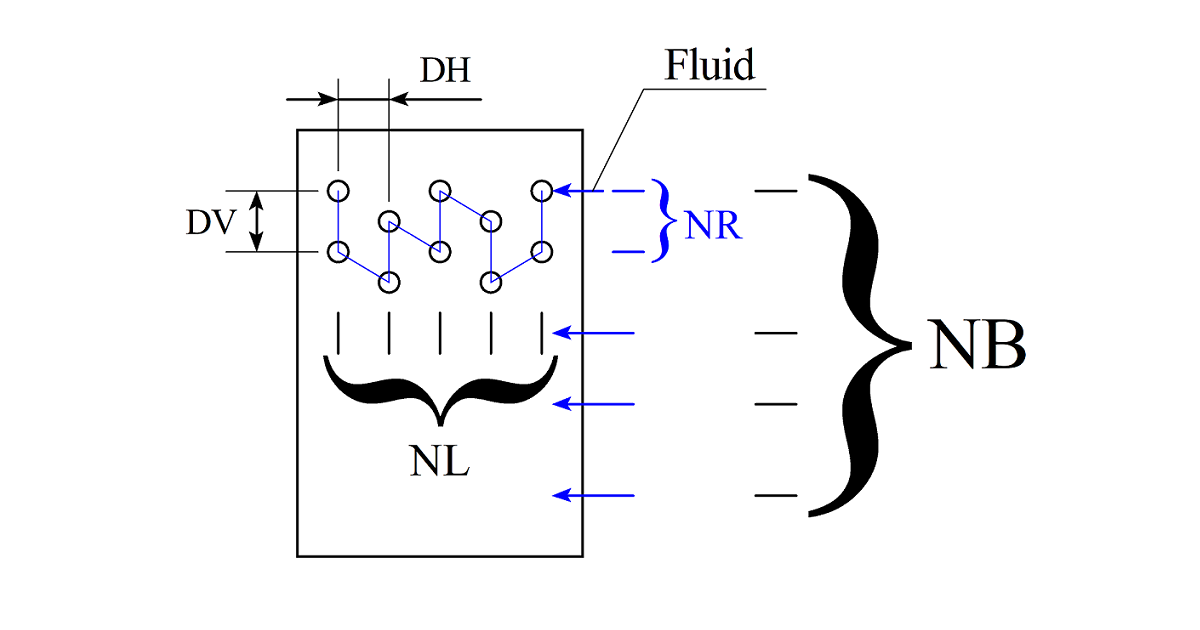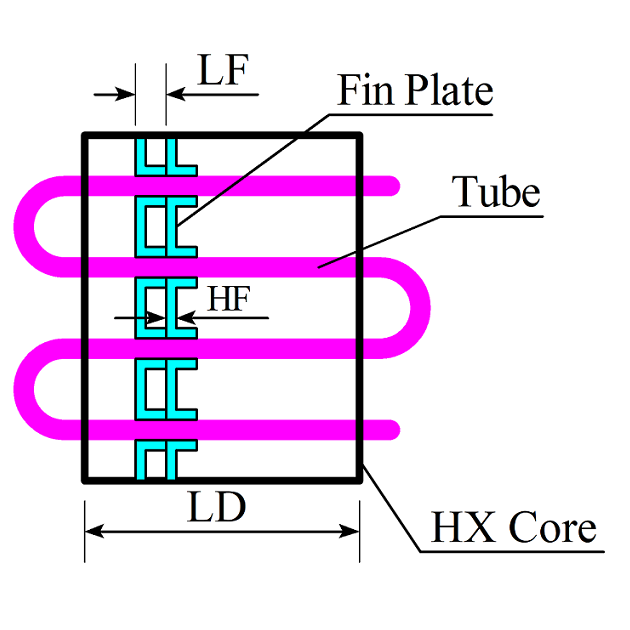#### Cooling/Heating Coil Design Calculation

...

Input Table
Quantity Value Unit
Fluid Selection
Fluid inlet flow kg/h
Fluid inlet pressure bar
Fluid inlet temperature °C
Inlet air flow rate kg/h
Inlet air pressure bar
Inlet air temperature °C
Inlet air humidity ratio g/kg(d.a)
Gas constant, 0=liquid, for manual input J/(kg*K)
Fluid density, for manual input kg/m3
Fluid specific heat, for manual input J/(kg*K)
Fluid dynamic viscosity, for manual input Pa*s
Fluid heat conductivity, for manual input W/(m*K)
Calculation mode (0=Design 1=Check)
Required cooling/heating load or heat transfer kW
Coil width initial mm
Tube outer diameter mm
Tube wall thickness mm
Fin spacing mm
Fin wall thickness mm
Row tube spacing mm
Face tube spacing mm
Air inlet velocity, 0=By flow input m/s
Circuits number
Rows along air flow
Number of tubes per circuit per row
Fin style(0=Straight 1=Slot 2=Triangle wave 3=Sine wave)
Fin material(-1=See notes 0=Aluminum 1=Copper 2=Steel)
Tube material(-1=See notes 0=Copper 1=Steel 2=Aluminum)
Arrangement, 0=In-line 1=Cross
In tube heat transfer enhancement
Air side heat transfer enhancement
In tube pressure drop enlargement
Air side pressure drop enlargement
Optimize object OB(0=Weight 1=Price/Cost)
Tube cost cost/kg
Fin cost cost/kg
Tube material density, for manual input kg/m3
Tube material conductivity, for manual input W/(m*K)
Fin material density, for manual input kg/m3
Fin material conductivity, for manual input W/(m*K)
Output Table
Quantity Value Unit
Fluid outlet pressure 0 bar
Fluid outlet temperature 0 °C
Air outlet flow 0 kg/h
Air outlet pressure 0 bar
Air outlet temperature 0 °C
Air outlet humidity ratio 0 g/kg(d.a)
Air outlet free water 0 g/kg(d.a)
Fluid flow resistance 0 Pa
Air flow resistance 0 Pa
Calculated coil width 0 mm
Coil thickness 0 mm
Coil height 0 mm
Calculated fluid side flow velocity 0 m/s
Calculated air side flow velocity 0 m/s
Calculated cooling/heating load or heat transfer 0 kW
Fluid Renaulds number 0
Air Renaulds number 0
Fluid side heat exchange area 0 m2
Air side heat exchange area 0 m2
Finned surface weighted efficiency 0 %
Fluid side heat exchange coefficient 0 W/(m2*K)
Air side heat exchange coefficient 0 W/(m2*K)
Logarithmic mean temperature difference 0 °C
Heat exchange effectiveness 0 %
Core fluid volume 0 L
Core fluid mass 0 kg
Weight/Cost (OB=0:kg 1:cost) 0 kg/cost
Notes
1. Material cost: use your local currency such as \$/kg
2. Tube and fin material: -1 = input physical properties at bottom
3. Calculation mode: Design = calculate the width from cooling load;
Check = calculate the cooling load from widthOnline Design Tools
 Unit Converter Online Psychrometric Chart Online p-H & T-s Diagram Condenser Coil Design Evaporator Coil Design Cooling/Heating Coil Design Tube Shell Heat Exchanger Design Capillary Design